Ex 3.4

Chapter 3 Class 10 Pair of Linear Equations in Two Variables (Term 1)
Serial order wise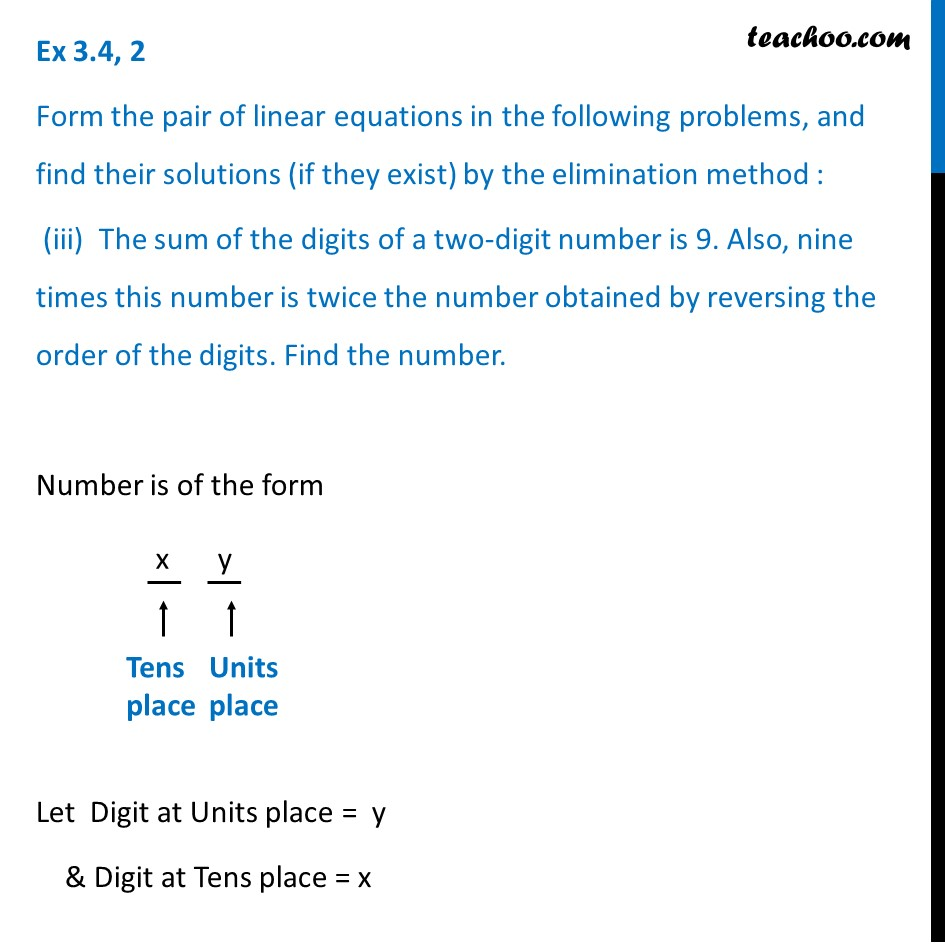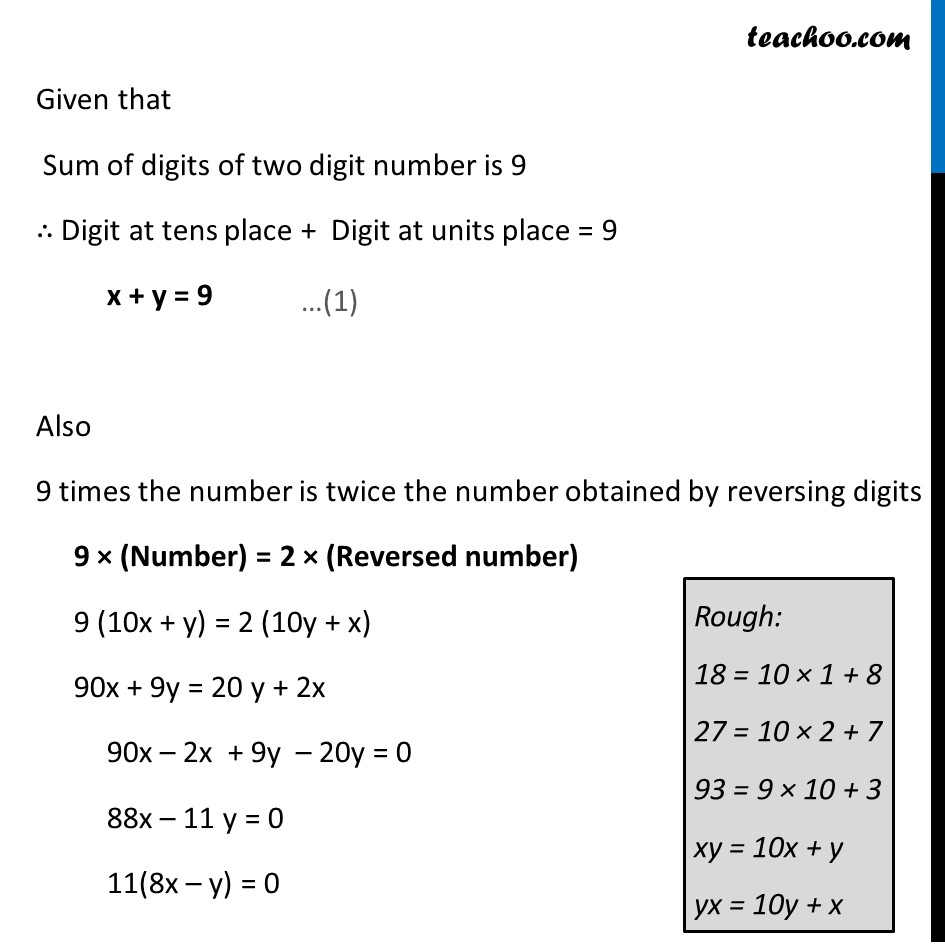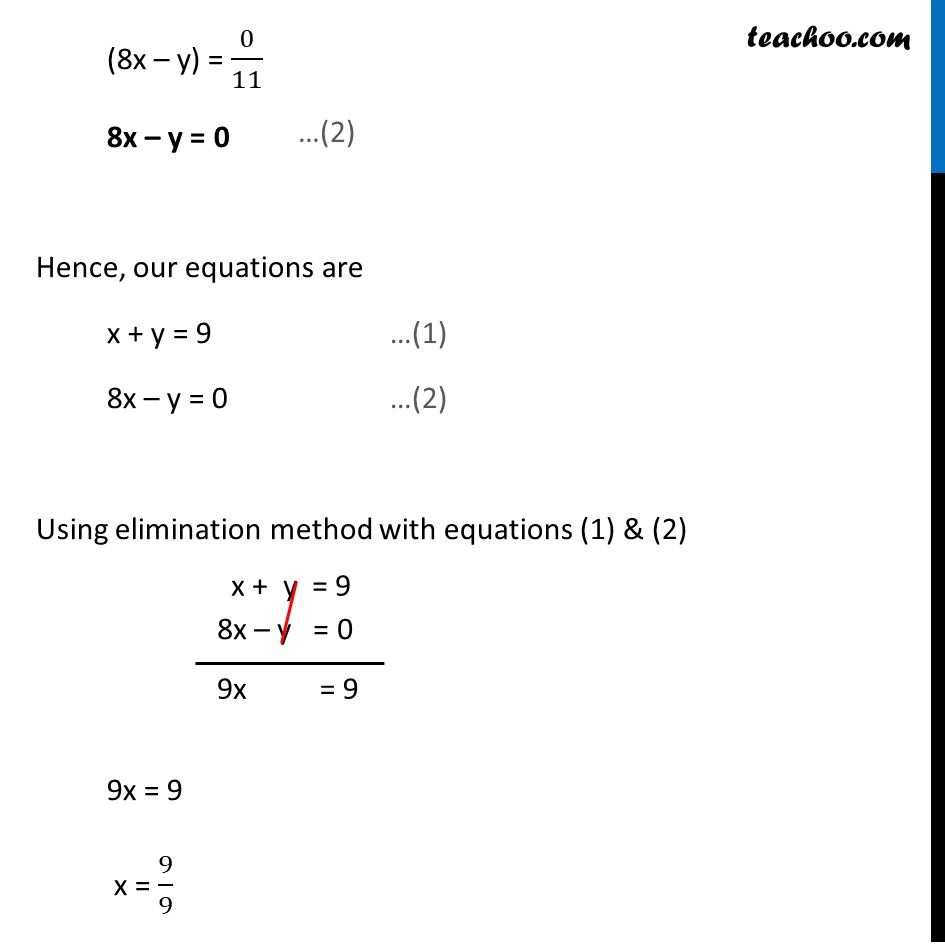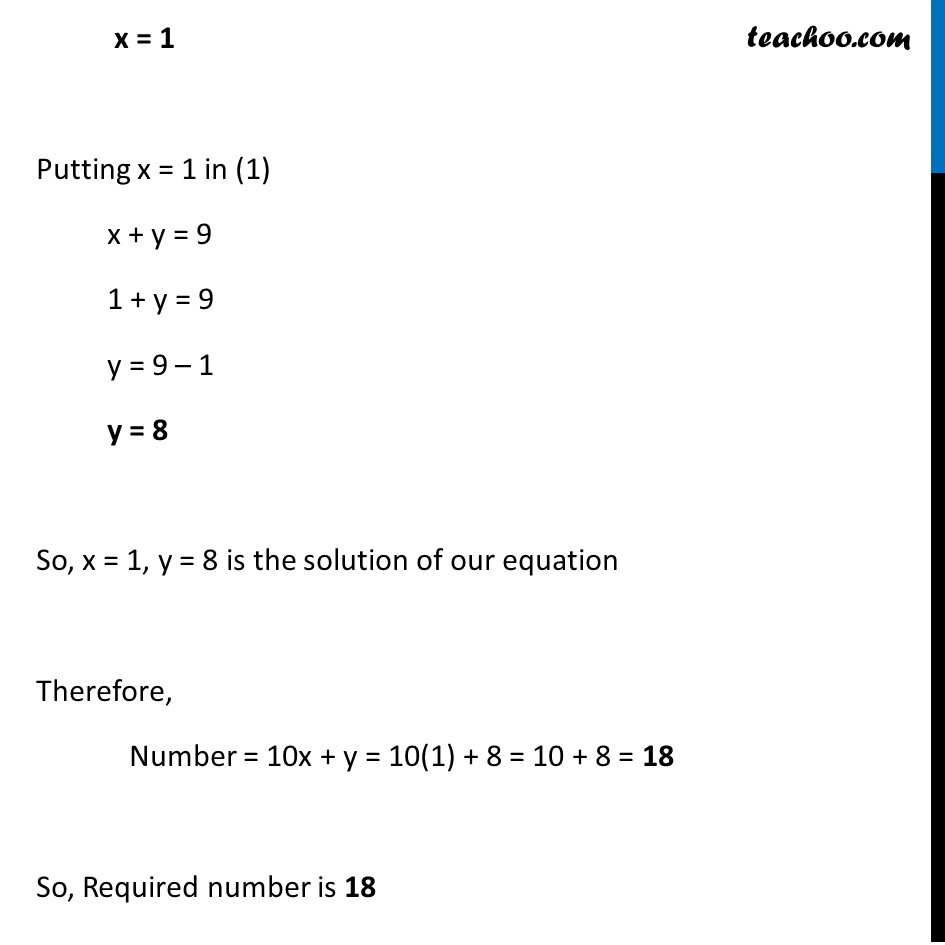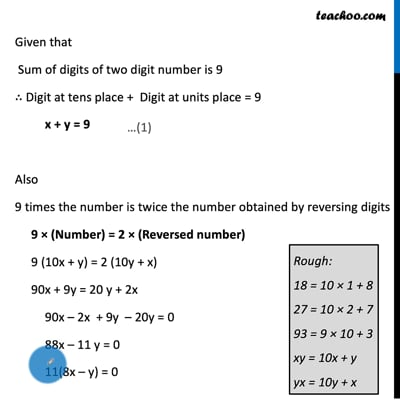This video is only available for Teachoo black users

### Transcript

Ex 3.4, 2 Form the pair of linear equations in the following problems, and find their solutions (if they exist) by the elimination method : (iii) The sum of the digits of a two-digit number is 9. Also, nine times this number is twice the number obtained by reversing the order of the digits. Find the number. Number is of the form Let Digit at Units place = y & Digit at Tens place = x Given that Sum of digits of two digit number is 9 ∴ Digit at tens place + Digit at units place = 9 x + y = 9 Also 9 times the number is twice the number obtained by reversing digits 9 × (Number) = 2 × (Reversed number) 9 (10x + y) = 2 (10y + x) 90x + 9y = 20 y + 2x 90x – 2x + 9y – 20y = 0 88x – 11 y = 0 11(8x – y) = 0 (8x – y) = 0/11 8x – y = 0 Hence, our equations are x + y = 9 …(1) 8x – y = 0 …(2) Using elimination method with equations (1) & (2) 9x = 9 x = 9/9 x = 1 Putting x = 1 in (1) x + y = 9 1 + y = 9 y = 9 – 1 y = 8 So, x = 1, y = 8 is the solution of our equation Therefore, Number = 10x + y = 10(1) + 8 = 10 + 8 = 18 So, Required number is 18